﻿ The Design of Robust Soft Sensor Using ANFIS Network

### The Design of Robust Soft Sensor Using ANFIS Network

Hamed Hosseini, Mehdi Shahbazian, Mohammad Ali TakassiOPEN ACCESSPEER-REVIEWED

## The Design of Robust Soft Sensor Using ANFIS Network

Hamed Hosseini1,, Mehdi Shahbazian1, Mohammad Ali Takassi2

1Department of Instrumentation and Automation engineering, Petroleum University of Technology, Ahvaz, Iran

2Department of Basic Sciences, Petroleum University of Technology, Ahvaz, Iran

### Abstract

A soft Sensor is a model which is used to estimate the unmeasurable output of an industrial process. Designing a soft sensor is usually difficult because its modeling is often based on case data. These data commonly contain the outliers and noise as soft sensor design is been problem. In order to solve the problem and successfully design a soft sensor, this paper introduces a new approach for designing a robust soft sensor which is not affected by outliers especially batch outlier and long tail noise. To response this goal, a robust soft sensor based on Adaptive Neuro-Fuzzy Inference System (ANFIS) which is based on robust cost function such as the summation of the absolute cost function. To minimize the cost function the particle swarm optimization (PSO) algorithm was used. The subtractive clustering technique was used to determine the ANFIS structure. The proposed method for designing a soft sensor is implemented on a chemical plant and compared with soft sensor based on ANFIS which is based on quadratic cost function. The simulation result shows higher accuracy in prediction of output variable in new robust soft sensor.

12345
Prev Next

### Cite this article:

• Hosseini, Hamed, Mehdi Shahbazian, and Mohammad Ali Takassi. "The Design of Robust Soft Sensor Using ANFIS Network." Journal of Instrumentation Technology 2.1 (2014): 9-16.
• Hosseini, H. , Shahbazian, M. , & Takassi, M. A. (2014). The Design of Robust Soft Sensor Using ANFIS Network. Journal of Instrumentation Technology, 2(1), 9-16.
• Hosseini, Hamed, Mehdi Shahbazian, and Mohammad Ali Takassi. "The Design of Robust Soft Sensor Using ANFIS Network." Journal of Instrumentation Technology 2, no. 1 (2014): 9-16.

 Import into BibTeX Import into EndNote Import into RefMan Import into RefWorks

### 1. Introduction

Soft (or inferential) sensors are a valuable tool in many different industrial fields of application. A soft Sensor is a model which is used to estimate the unmeasurable output of an industrial process. The expression soft sensor is mainly used in the field of process industry, but the utility of mathematical modelling is widespread in engineering fields.

A first wave of soft sensors based on the “classical” back- propagation neural network approach is in use in different areas of manufacturing since the early 1990’s . Studies of use of soft sensors that mostly focus to process industry can be found in [2, 3, 4]. The approach is however not limited to process industries and similar ideas are utilized in other engineering fields, for example in traffic engineering, e.g. [5, 6]. Soft-sensors are used where their hardware counterparts are not available, are very costly or their installation is very costly.

In general Soft-sensors can be divided to two different classes which are model-driven and data-driven Soft-sensors. The model-driven family of Soft-sensors is most commonly based on theoretical or so called first principle models or on extended Kalman filter. The data-driven family of Soft-sensors is based on data measured within the systems. The data-driven Soft-sensors gained increasing popularity in the process industry, because data-driven models are built on the data measured within the processing plants and describe the real process situations.

Soft-sensors are based on process model that is obtained from measured data. Most real-world databases include a certain amount of exceptional values, generally termed as “outliers”. Presence of outliers in training and testing data can bring about several difficulties for learning methods. The isolation of outliers is important both for improving the quality of original data and for reducing the impact of outlying values in the process of knowledge discovery in databases. Thus, removing or replacing outliers can improve the quality of stored data. Isolating outliers may also have a positive impact on the results of data analysis and data mining.

Measured data need a lot of pre-processing due to: missing data, data outliers, drifting data, data co-linearity, different sampling rates and measurement delays. Robustness toward process variability, industrial data quality, and insufficient modeling are key subjects for reliable and mass-scale application of inferential Soft-sensors.

The most popular modelling methods for data-driven Soft-sensors are principle component analysis (PCA), partial least squares, artificial neural networks, Neuro-Fuzzy systems and support vector machines . Hybrid methods are popular for designing of data-driven soft sensor. Among these methods Neuro-Fuzzy has gained a high popularity within the past few years due to its benefits over other methods. A well-known Neuro-Fuzzy model is the ANFIS model . ANFIS is an architecture which functionally integrates the interpretability of a fuzzy inference system with adaptability of a neural network. In other words, ANFIS is a method for tuning an existing rule base of fuzzy system with a learning algorithm based on a collection of training data found in artificial neural network. The ANFIS composes the ability of Neural Network and Fuzzy System.

Most of the widely used training methods for determining ANFIS parameters are gradient based. The outliers reduce the quality of the data and affect the derivation calculation accuracy required. This makes the methods to be not always robust. A novel training approach based on summation of absolute cost function has been proposed to overcome the shortcoming. This function is able to train and update the ANFIS parameters and reduces the effects of the outliers. The PSO, a gradient free based optimization algorithm, also has been employed to consider the absolute cost function requirements and find the solution fast.

The subtractive clustering technique was used to determine the ANFIS structure. The rest of paper is organized as follows: In section 2 a full description of the method is proposed. The implementing and its results of the purposed method on a simulated chemical plant together with a short description of the plant are presented in section 3. The paper is terminated with the conclusion in section 4.

### 2. Methodology

2.1. Adaptive Neuro-Fuzzy Inference System (ANFIS)

Here, in this section, we present the basic theory of ANFIS model. ANFIS is a multi-layer adaptive network-based fuzzy inference system proposed by Jang . In this paper, an ANFIS is presented as the Neuro-Fuzzy systems is subtractive clustering based fuzzy inference system, named Sub.Clustering ANFIS. The network applies a combination of the least square method and the back propagation gradient descent method for training ANFIS to imitate a given training data set. When the training and testing errors are within the acceptable bound, therefore the system converges. This construction has demonstrated high performance in many applications.

A typical ANFIS construction is shown in Figure 1. The circular nodes represent nodes that are fixed whereas the square nodes are nodes that have parameters to be learnt. For simplicity, consider two inputs (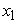and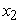), one output (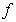). The Sugeno model is formed by using five layers and two if–then rules.

ANFIS’s system architecture has five layers. The details of the algorithm are explained below:

Layer 1. Every node i in this layer is an adaptive node with a node function.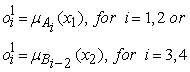(1)

Where x1 (or x2) is the input to node i and Ai (or Bi-2) is a linguistic label associated with this node. Here the membership function forA1, A2, B1 or B2 can be any appropriate parameterized membership function, like for example the generalized bell membership function: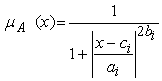(2)

Where ai, bi and ci are the parameters of membership functions. Parameters in this layer are referred to as premise (antecedent) parameters.

Layer 2. Every node in this layer is a fixed node, whose output is the product of all the incoming signals:

Each node output represent the firing strength of a rule. In general, any other T-norm operators that perform fuzzy and can be used as the node function in this layer.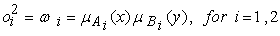(3)

Layer 3. Every node in this layer is a fixed node labelled N. The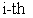node calculates the ratio of therules firing strength to the sum of all rules firing strengths: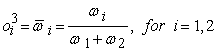(4)

For suitability, outputs of this layer are called normalized firing strengths.

Layer 4. Every node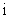in this layer is an adaptive node with a node function: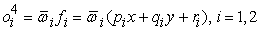(5)

Where pi, ri and qi are the design parameters. Parameters in this layer are referred to as consequent parameters.

Layer 5. The single node in this layer is a fixed, which the overall output is computed by the summation of all incoming signals .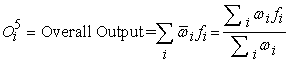(6)
2.2. Subtractive Clustering

The subtractive clustering method is proposed by Chiu . Clustering data set in an unsupervised method by measuring the potential of data set in the feature space is subtractive clustering technique. Subtractive clustering assumes that each data point is a potential cluster center and calculates the potential for each data point based on the density of surrounding data points. The first cluster center has the highest potential and the potential of data points near the first cluster center (within the influential radius) is destroyed. The radius of influence is important to determine the number of clusters. A smaller radius leads to many smaller clusters in the data space, which results in more rules, and vice versa. It is important to choice suitable radius of influence for clustering the data space. The Subtractive Clustering technique is used to determine the ANFIS structure. Subtractive Clustering looks for an optimal data point by distributing the data into clusters and defining a cluster center based on the density off surrounding data points. The Subtractive Clustering algorithm has the following steps:

1. Select the data point with the highest potential to be the first cluster center.

2. All data points in the vicinity of the first cluster center (as determined by radii) are removed in order to determine the next data cluster and its center location.

3. This process is iterated until all of the data is within radii of a cluster center.

The optimal point defining a cluster center is found with proper cluster radii .

2.3. The Outliers

Outliers are the cases with data values different than the values of the majority of the cases in the data set. Outliers are patterns in data that do not conform to a well-defined no notion of normal behavior. Figure 2 illustrates outliers in a simple 2-dimensional data set. The data has two types of outliers (single/batch).

Points that show sufficiently far away from the data set, are outliers . In analytical chemistry, empirical data often contain outliers of one type (Single Outlier) or another (Batch Outlier). Statistical techniques are often used which are sensitive to such outliers, and negative results may have been affected by them, and the most robust and resistant methods have been developed since 1960 and less sensitive to outliers. Robustness is the key issue for modeling and identification.

2.3. Absolute cost function as robust cost function

Robust Cost Functions are alternative cost functions which are robust against outliers and noise. Quadratic cost function is the conventional cost function which is used as optimization tool. Figure 3 show the Quadratic cost function.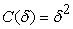(7)

Absolute cost function is a type of robust cost function. Properties of Absolute cost function are absolute error, called ‘total variation’, convex and non-differentiable at origin. Figure 4 show the Absolute cost function.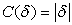(8)
2.4. Particle Swarm Optimization (PSO) Algorithm

This section gives a brief over view of PSO method. Also, the procedure of the minimization of the robust cost function by using the PSO algorithm for the solution is given.

Particle Swarm Optimization (PSO) is a method used to explore the search space of a given problem to ﬁnd the settings or parameters required to maximize a particular objective. This method, ﬁrst described by James Kennedy and Russell C. Eberhart in 1995 , originates from two separate concepts: the idea of swarm intelligence based oﬀ the observation of swarming habits by certain kinds of animals such as birds and ﬁsh and the ﬁeld of evolutionary computation. It uses a number of particles that constitute a swarm moving around in the search space looking for the best solution. Each particle is treated as a point in a N-dimensional space which adjusts its “flying” according to its own flying experience as well as the flying experience of other particles.

Each particle keeps track of its coordinates in the solution space which are associated with the best solution (fitness) that has achieved so far by that particle. This value is called personal best (pbest).

Another best value that is tracked by the PSO is the best value obtained so far by any particle in the neighborhood of that particle. This value is called global best (gbest).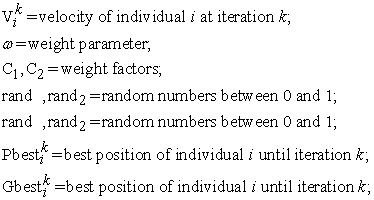In the PSO algorithm, the velocity of particle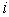is updated according the following equation: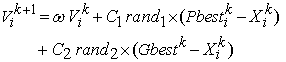(9)

Each individual moves from the current position to the next one by the modified velocity in (9) using the following equation: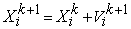(10)

Using the modified velocity and position of particlebased on (9) and (10), the search mechanism of the PSO is demonstrated in Figure 5.

The general PSO algorithm can be summarized as follows:

Initialize a population (array) of particles with random positions and velocities of N-dimensions in the problem space.

Evaluate the desired optimization fitness function in N variables for each particle.

Compare particle's fitness evaluation with particle's pbest. If current value is better than pbest, then set pbest value equal to the current value and the Pbest location equal to the current location in d-dimensional space.

Compare fitness evaluation with the population's overall previous best. If the current value is better than Gbest, then reset Gbest to the current particle's array index and value.

Change the velocity and position of the particle according to (9) and (10) respectively. Vi and Xi represent the velocity and position of ith particle with N-dimensions respectively and rand1 and rand2 are two uniform random functions.

Go to Step 2 until satisfying stopping criteria, usually a sufficiently good fitness or a maximum number of iterations / epochs .

PSO method has many advantages; it is simple, fast and easy to be coded. Another advantage is that the initial population of the PSO is maintained, and so there is no need for applying operators to the population, a process that is time and memory-storage-consuming.

2.5. Selection of Parameters for PSO Algorithm

The selection of these PSO parameters plays an important role in the optimization . A single PSO parameter choice has a great effect on the rate of convergence. For this paper, the optimal PSO parameters are determined by trial and error experimentations.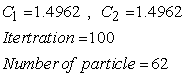A smaller radius leads to many smaller clusters in the data set space, which results in more rules, and vice versa. Hence it is important to select suitable influential radius for clustering the data set space.

The optimum radius, (0.6), is determined by trial and error experimentations.

2.6. Training ANFIS with PSO

In this section, the PSO method employed for minimization of robust cost function in order to update the parameters of ANFIS structure. The ANFIS has two types of parameters which need to update, the antecedent parameters and the conclusion parameters. The membership functions are assumed Gaussian as in equation (11), and their parameters are Ci and σi, where σi is the variance of membership functions and Ci is the center of membership functions.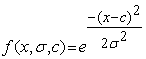(11)

The parameters of conclusion part are represented with pi, ri and qi that are shown in (12) and (13).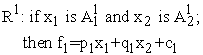(12)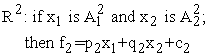(13)

### 3. Case Study, Implementation and Results

3.1. Case Study

A model of a highly nonlinear chemical plant consisting of two cascade Continuous Stirred Tank Reactors (CSTRs) followed by a no adiabatic flash separator with a recycle has been selected in this paper .

A simple graph of the plant is displayed in Figure 6. This combination is prevalent in chemical industries such as the styrene polymerization process. The desired product B is produced by an irreversible first order reaction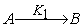.

An undesirable side reaction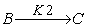occurs and leads to the consumption of B and the production of the superfluous side product C.

The product stream from CSTR-2 is directed into a flash to separate the excess A from the product B and the side product C. Reactant A has the highest relative volatility and is the major component in the vapor phase. A fraction of the vapor phase is purged and the residual stream is condensed and recycled back to CSTR-1. The liquid phase exiting from the flash consists predominantly of B and C.

In order to test the ability of soft sensor to track the process changes, we define some realistic scenarios of changes on the plant as follow:

1. A step change in the concentration of the input feed. At first, it is supposed that the input feed purely consists of component A. After the application of the step change, the input feed contains both components A and B with fractions of 90% and 10%, respectively.

2. Random changes in Qr, T0, D and F0 each with an amplitude of about 10% of its operating point value. The changes happen slowly and randomly, which is closer to the real case events.

All the changes are applied to the model during the test stage.

The inputs of soft sensor are: D, F0, F1, Hb, Hm, Hr, Qb, Qm, Qr, T0, Tb, Td, Tm and Tr which can easily be measured and the output is the product mass fraction XBb.

As it is mentioned before, the proposed approach has the ability of robust training. It means that the proposed approach will reduce the influence of noise and outliers on the performance of the soft sensor.

The model is implemented in Simulink. We generate about 600 samples from the model. All the variables of training set are scaled to [0, 1]. 240 data points are used as the training set and 360 data points are used for testing the model.

3.2. Performance Indices

In this study, in addition to graphical demonstration we use two common numerical measures for performance evaluation. The Root Mean Square Error (RMSE) (also called the Root Mean Square Deviation, RMSD) is a frequently used measure of the difference between values predicted by a model and the values actually observed from the environment that is being modelled. These individual differences are also called residuals, and the RMSE serves to aggregate them into a single measure of predictive power.

The RMSE of a model prediction with respect to the estimated variable Xmodel is defined as the square root of the mean squared error: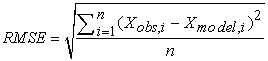(14)

where Xobs is observed values and Xmodel is modelled values at time/place i.

The calculated RMSE values will have units, and RMSE for phosphorus concentrations can for this reason not be directly compared to RMSE values for chlorophyll a concentrations etc. However, the RMSE values can be used to distinguish model performance in a calibration period with that of a validation period as well as to compare the individual model performance to that of other predictive models.

RMSE demonstrates how well the predicted output fits the true output but it does not necessarily reflect whether the two sets of data move in the same direction. For instance, by simply scaling the system output, we can change the RMSE without changing the directionality of data. The correlation coefficient (R) solves this problem. The correlation coefficient is calculated by: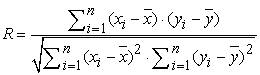(15)

The correlation coefficient tells us the strength and direction of the relationship between two variables. The closer the number is to 0, the weaker the relationship. The closer the number is to ±1.00, the stronger the relationship. Therefore, the R lies in the range [-1, 1]. When R = 1, there is a perfect positive linear correlation between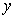and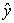, that is they vary by the same amount. When R = -1 there is a perfectly linear negative correlation betweenand, that is they vary in opposite ways (whenincreases,decreases by the same amount). When R = 0 there is no correlation between,and the variables are called uncorrelated. Intermediate values describe partial correlations.

3.3. Implementation of the methodology

At the first stage of the purposed algorithm, we generate a structure ANFIS by subtractive clustering. The first-order Sugeno fuzzy model was used in consequence part. In addition, in order to reduce the number of parameters to be optimized by the robust training method in the ANFIS structure, only two parameters have been used for the Gaussian membership functions in the premise part of each rule. Then use absolute cost function as robust cost function to updates the parameters of this structure. We employed PSO algorithm for minimization of cost functions.

After training step, for evaluating the performance of the trained ANFIS model and to monitor how well the network is training, the test data sets were presented to the network.

In this study, in addition to graphical demonstration we use two common numerical measures for performance evaluation: The Root Mean Square Error (RMSE) and correlation coefficient (R).

RMSE demonstrates how well the predicted output fits the true output but it does not necessarily reflect whether the two sets of data move in the same direction and the correlation coefficient (R) gives more information about the training of network.

The results of using quadratic and absolute cost function for training ANFIS are shown in the following table and also the plot of the training data, testing data and correlation coefficient (R) for each method is displayed.

According to this study the results of the new method are very close to the experimental results and the correlation coefficient (R) value is 0.88128 for testing data sets.

The stopping criterion is the number of iterations as the maximum iterations is 100.

3.4. Results and discussion

The simulation result show a very higher prediction accuracy in comparison with the ANFIS method for designing soft sensor. Figure 7 shows data set of soft sensor. Figure 8, Figure 9 and Figure 10 show the results Train data, Test data and Correlation coefficient of ANFIS approach and Figure 11, Figure 12 and Figure 13 show the results Train data, Test data and Correlation coefficient of new method for designing soft sensor. The results of using quadratic and robust cost function for training ANFIS are shown in the Table 1. As is shown in Figures and Table 1, a new method for the design of the soft sensor is robust against Gaussian noise and single/batch outliers, while other methods such as ANFIS and neural network are strongly affected by Gaussian noise and single/batch outliers. The correlation coefficient is closer to one, the better for us. Mean square error is used as quantitative measure for performance evolution of the training method.

Figure 13. Correlation coefficient of the proposed robust approach for CSTR data set

#### Table 1. Results (RMSE and Correlation Coefficient)Download asPowerPoint Slide

Veiw figureView current table in a new window

### 4. Conclusion

This paper presents a new approach to design a robust soft sensor against noise and outliers. In this approach, ANFIS structure has been used and the PSO algorithm is used to minimization of cost function. Summation of the absolute cost function has been used as cost function in this method. The training method of ANFIS parameters can have a huge effect on the accuracy of identification and prediction. So, we can use PSO as a new tool for training ANFIS network that result in reduction of complexity.

Due to this study the new purposed method for designing a soft-sensors is more robust in the presence of noise and outliers.

### References

  R. Neelakantan. and J. Guiver., “Applying Neural Networks,” Hydrocarbon Processing, vol. 9, pp. 114-119, 1998.In article  P. Kadlec., B. Gabrys., and S. Strandt., “Data-driven Soft Sensors in the process industry,” Computers and Chemical Engineering, vol. 33, pp. 795-814, 2009.In article CrossRef  G. D. Gonzalez., “Soft sensors for processing plants,” in Proceedings of the second international conference on intelligent processing and manufacturing of materials, IPMM99, 1999.In article  L. Fortuna., S. Graziani., A. Rizzo., and M. G. Xibilia, “Soft Sensors for Monitoring and Control of Industrial Processes,” London: Springer-Verlag, 2007.In article  A. Adamski. and S. Habdank-Wojewodzki., “Traffic congestion and incident detector realized by fuzzy discrete dynamic system,” Archives of Transport, vol. 17, pp. 5-13, 2004.In article  W. B. Zhu., D. S. Li., and Y. Lu., “Real time speed measure while automobile braking on soft sensing technique,” Journal of Physics: Conference Series, vol. 48, pp. 730-733, 2006.In article  J. S. R. Jang., “ANFIS: Adaptive-network-based fuzzy inference systems,” IEEE Trans, Syst, Man Cybern, vol. 23, pp. 665-685, 1993.In article CrossRef  S. Chiu., “Fuzzy Model Identification Based on Cluster Estimation,” Intelligent & Fuzzy Systems, vol. 2, 1994.In article  R. Kothandaraman. and L. Ponnusamy., “PSO tuned Adaptive Neuro-fuzzy Controller for Vehicle Suspension Systems,” Journal of Advances in Information Technology, vol. 3, pp. 57-63, Feb 2012.In article CrossRef  K. Singh. and S. Upadhyaya., “Outlier Detection: Applications And Techniques” International Journal of Computer Science Issues, vol. 9, pp. 307-323, January 2012.In article  J. Kennedy. and R. Eberhart., “Particle Swarm Optimization,” Proceedings of IEEE International Conference on Neural Networks IV., pp. 1942-1948, 1995.In article CrossRef  Y. Shi and R. Ebcrhart, “Paramctcr Sclcction in Panicle Swarm Optimization,” Proe. Scvcnth Annual Conf. on Evolutionary Programming, pp. 591-601, March 1998.In article CrossRef  S. Kamelian., “Adaptive distributed control of industrial plants using stability-based nonlinear technique,” Ahwaz, 2012.In article